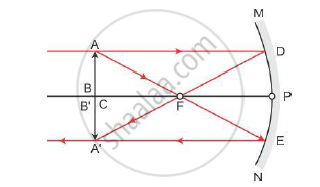# It is desired to obtain an erect image of an object, using concave mirror of focal length of 12 cm. Where will the image of this object be, if it is placed 24 cm in front of the mirror? - Science

It is desired to obtain an erect image of an object, using concave mirror of focal length of 12 cm.

Where will the image of this object be, if it is placed 24 cm in front of the mirror? Draw ray diagram for this situation also justify your answer. Show the positions of pole, principal focus and the centre of curvature in the above ray diagrams

#### Solution

Since f = 12 cm  Centre of curvature = 2f = 24 cm
For an object placed at a distance 24 cm, i.e. at the centre of curvature of a concave mirror, the image formed will be real, inverted and of the same size as that of the objectConcept: Concave Mirror
Is there an error in this question or solution?